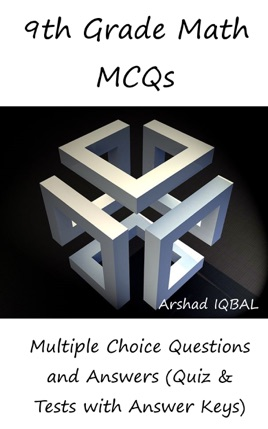• \$5.99

## Publisher Description

9th grade math multiple choice questions has 540 MCQs. Grade 9 math quiz questions and answers pdf, MCQs on Cartesian plane, linear graphs, characteristics of logarithm, collinear points, common and natural logarithms, complex numbers, congruent triangles, construction of triangles, conversion graphs, cumulative frequency, MCQs on de Morgan’s law, distance formula, equations and absolute value, factorization, frequency distribution, geometric mean, geometric sequence, sets MCQs and quiz are to practice exam prep tests.
9th grade math multiple choice quiz questions and answers pdf, math exam revision and study guide with practice tests for online exam prep and interviews. Math interview questions and answers to ask, to prepare and to study for jobs interviews and career MCQs with answers keys.
Algebraic expressions and algebraic formulas quiz has 49 multiple choice questions. Algebraic manipulation quiz has 13 multiple choice questions. Arithmetic and geometric sequences quiz has 36 multiple choice questions. Basic math problems quiz has 20 multiple choice questions. Business mathematics quiz has 37 multiple choice questions. Congruent triangles and geometry quiz has 17 multiple choice questions.
Basic statistics quiz has 35 multiple choice questions. Consumer math quiz has 20 multiple choice questions. Factorization quiz has 13 multiple choice questions and answers. Introduction to logarithms quiz has 30 multiple choice questions. Linear equations and inequalities quiz has 27 multiple choice questions and answers. Linear graphs and applications quiz has 16 multiple choice questions with answers. Logarithms and exponents quiz has 12 multiple choice questions.
Mathematical theorems quiz has 24 multiple choice questions. Matrices and determinants quiz has 48 multiple choice questions and answers. Percentage, ratio and proportion quiz has 51 multiple choice questions. Real and complex numbers quiz has 61 multiple choice questions. Sets and functions quiz has 110 multiple choice questions.
Math jobs' interview questions and answers pdf, MCQs on addition and subtraction of matrices, algebraic expressions, algebraic formulae, area of figure, arithmetic mean, arithmetic sequence, binary relation, business partnership, Cartesian plane and linear graphs, characteristics of logarithm, collinear points, common logarithm and natural logarithm, complex numbers, congruent triangles, construction of triangles, conversion graphs, cumulative frequency, de Morgan’s law, discount formula, distance formula, equations involving absolute value, factorization, frequency distribution, geometric mean, geometric sequence, important sets, introduction to logarithms, introduction to ratio and proportion, L.C.M and H.C.F, laws of logarithm, linear equations, linear inequalities, logarithms, math theorems, mathematical definitions, mathematical ratios, matrix, measures of central tendency, measures of dispersion, mid-point formula, multiplication of matrices, multiplicative inverse, multiplicative inverse of matrix, operations on sets, ordered pairs, percentage calculations, personal income, profit and loss, properties of real numbers, proportions in math, Pythagoras theorem, radicals and radicants, rationalization of surds, real numbers, rectangular region, remainder theorem and factor theorem, scientific notation, sequence, sets, solution of simultaneous linear equations, solving linear inequalities, square root of an algebraic expression, surds and their application, taxes, triangular region, types of matrices, Math worksheets for competitive exams preparation.